Function Repository Resource:

# CurvedSpacetimeSprinkling

Produce random causal graphs by sprinkling points into a spacetime with a specified algebraic curvature function

Contributed by: Jonathan Gorard
 ResourceFunction["CurvedSpacetimeSprinkling"][func,{x,xmin,xmax},n] produces a random sprinkling of n points into a one-dimensional spacetime with algebraic curvature given by func as a function of x. ResourceFunction["CurvedSpacetimeSprinkling"][func,{x,…},{y,…},…] produces a random sprinkling of n points into a higher-dimensional spacetime with multivariable algebraic curvature function func (in variables x,y,…, etc.). ResourceFunction["CurvedSpacetimeSprinkling"][func,{x,…},…,n,"prop"] gives the property "prop" for the spacetime sprinkling with the specified algebraic curvature function.

## Details and Options

ResourceFunction["CurvedSpacetimeSprinkling"][func,{x,},,n] returns a SpacetimeSprinkling object by default.
Unlike the resource function FlatSpacetimeSprinkling, sprinklings can only be performed in 0+1-, 1+1- and 2+1-dimensional spacetimes using ResourceFunction["CurvedSpacetimeSprinkling"].
Note that, in the multivariable case, only algebraic curvature functions are supported.
Sprinklings are performed using a Poisson point process, with the expected number of sprinkled points being proportional to the spacetime region volume so as to guarantee Lorentz invariance.
Causal edges are added in accordance with the standard causal partial order relation on spacetime (as determined using the usual form of the spacetime line element) and are transitively reduced by default.
CurvedSpacetimeSprinking[func,{x1,},,{xm,},] treats the last variable, xm, as being the time coordinate with the other xi being the spatial coordinates.
ResourceFunction["CurvedSpacetimeSprinkling"] uses a similar argument specification to ProbabilityDistribution.
In ResourceFunction["CurvedSpacetimeSprinkling"][func,{x,},,n,"prop"], the following properties can be requested:
 "CausalGraphFull" full causal partial order graph (before transitive reduction) "CausalGraph" transitively reduced causal partial order graph "Points" plot of the sprinkled points only (without causal edges) "PointsList" list of the spacetime coordinates of all sprinkled points "Dimensions" number of dimensions in the continuum spacetime approximation "TotalEdgeCount" total number of causal edges (before transitive reduction) "ReducedEdgeCount" number of transitively reduced causal edges "PureCausalGraphFull" full causal partial order graph (before transitive reduction) with vertex coordinate information removed "PureCausalGraph" transitively reduced causal partial order graph with vertex coordinate information removed "Properties" list of properties
The sprinkling of elements of a discrete causal partial order into a continuous Lorentzian manifold via a Poisson point process was first described in the context of causal set theory by Sorkin, Bombelli and Henson.

## Examples

### Basic Examples (3)

A two-variable algebraic curvature function:

 In:=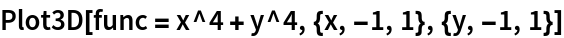Out=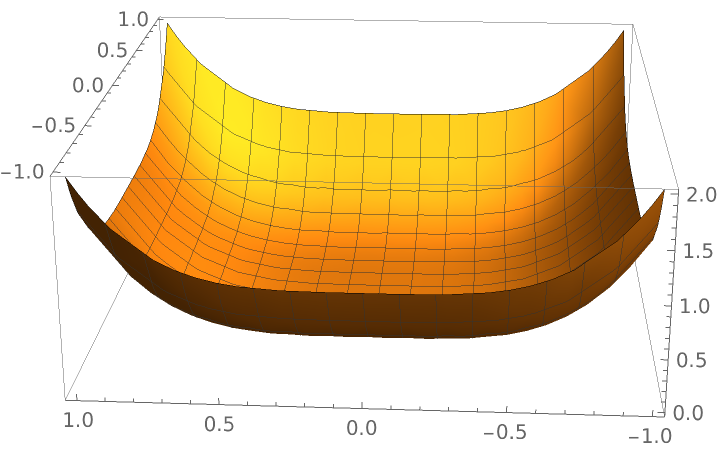Produce a random sprinkling of 100 points into a 1+1-dimensional spacetime with the specified curvature function:

 In:=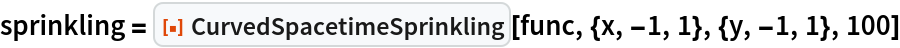Out=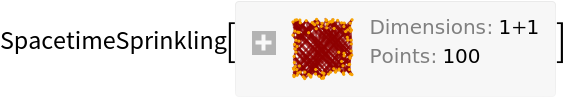Show the causal graph:

 In:=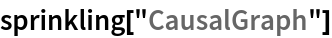Out=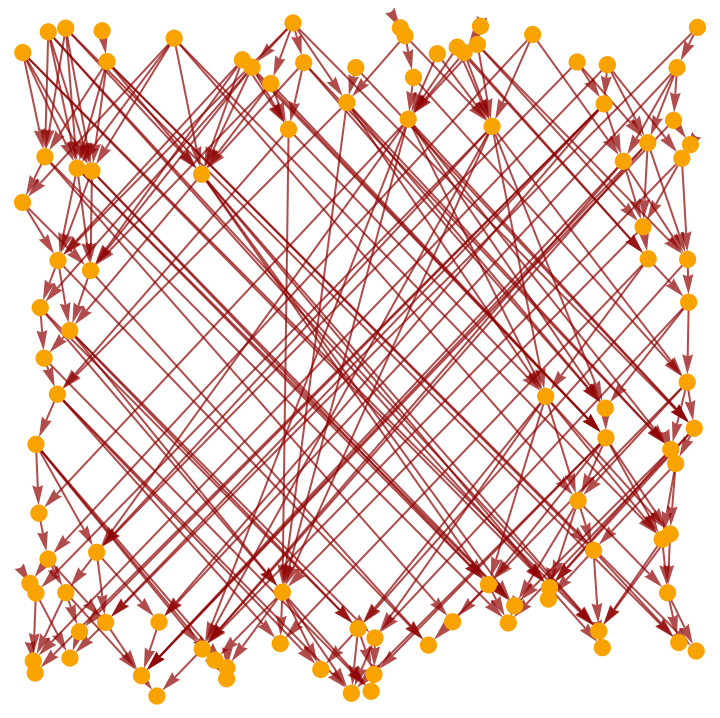Show the full causal graph (without transitive reduction):

 In:=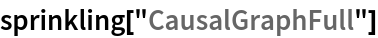Out=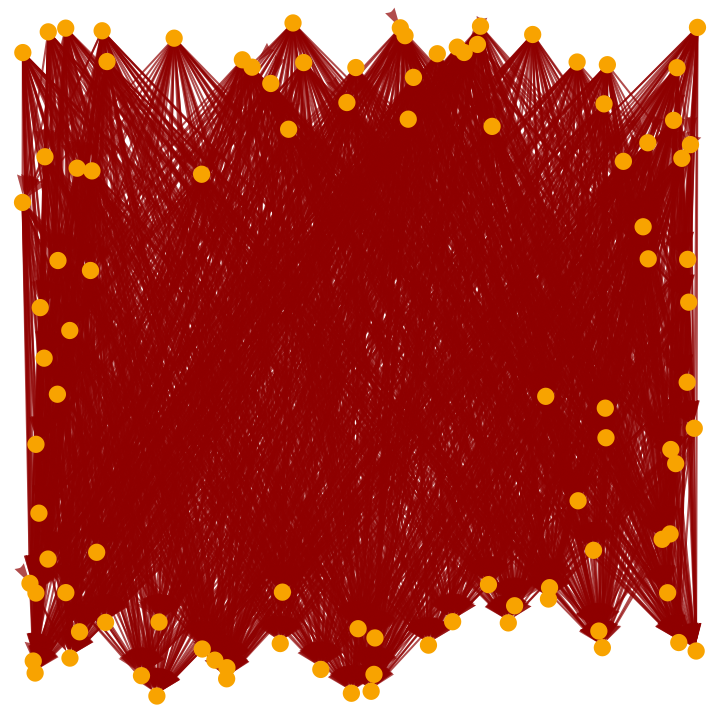A slightly more complicated two-variable algebraic curvature function:

 In:=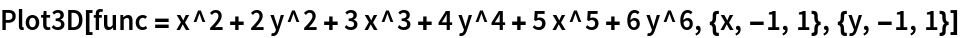Out=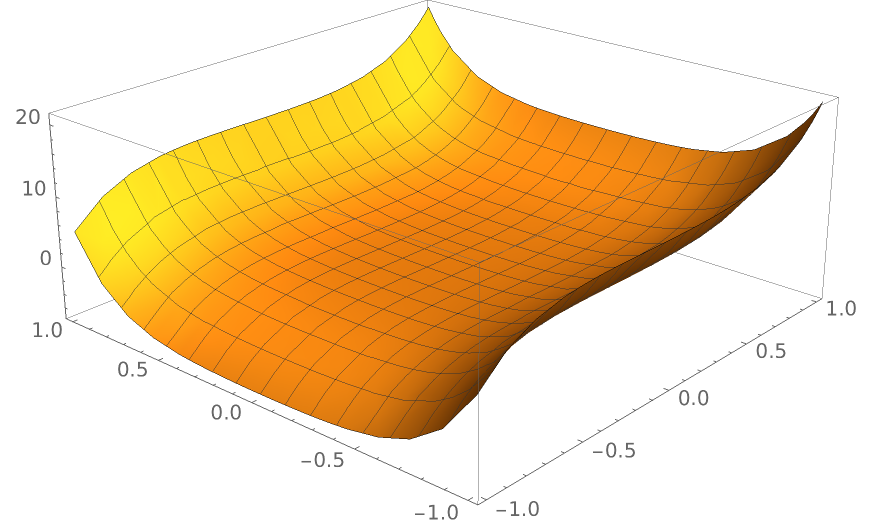Produce a random sprinkling of 200 points into a 1+1-dimensional spacetime with the specified curvature function:

 In:=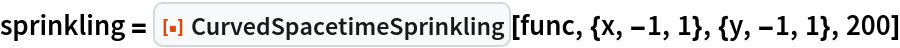Out=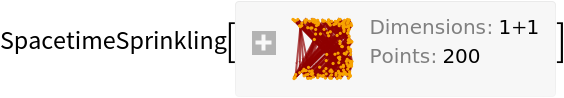Show the causal graph:

 In:=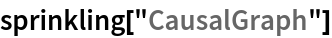Out=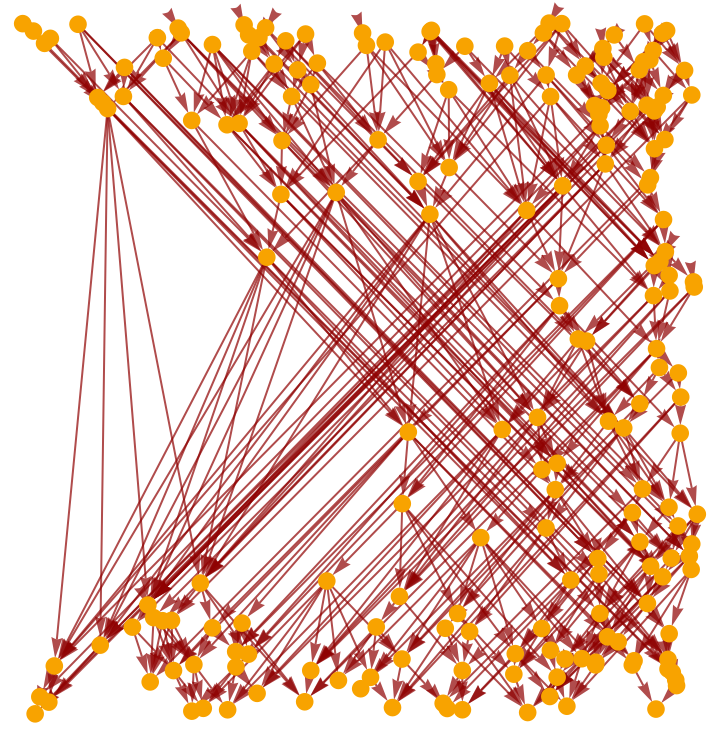Show the pure causal graph (with vertex coordinate information removed):

 In:=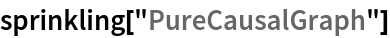Out=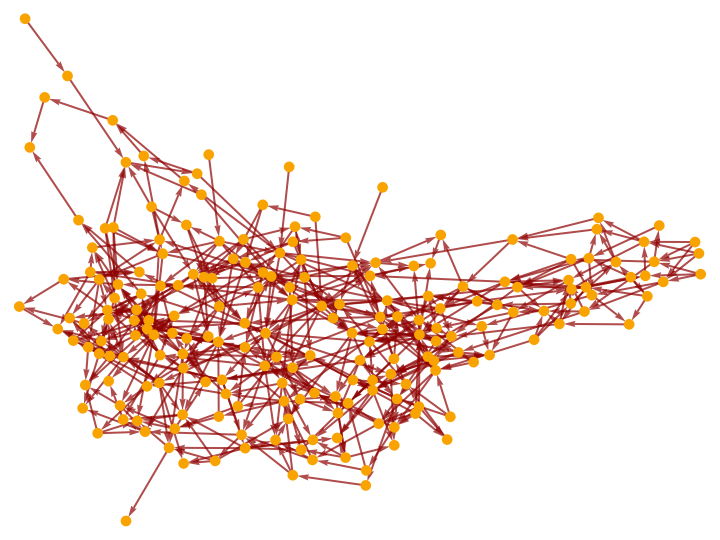Show the pure causal graph with a layered graph embedding:

 In:=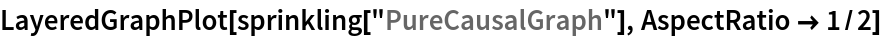Out=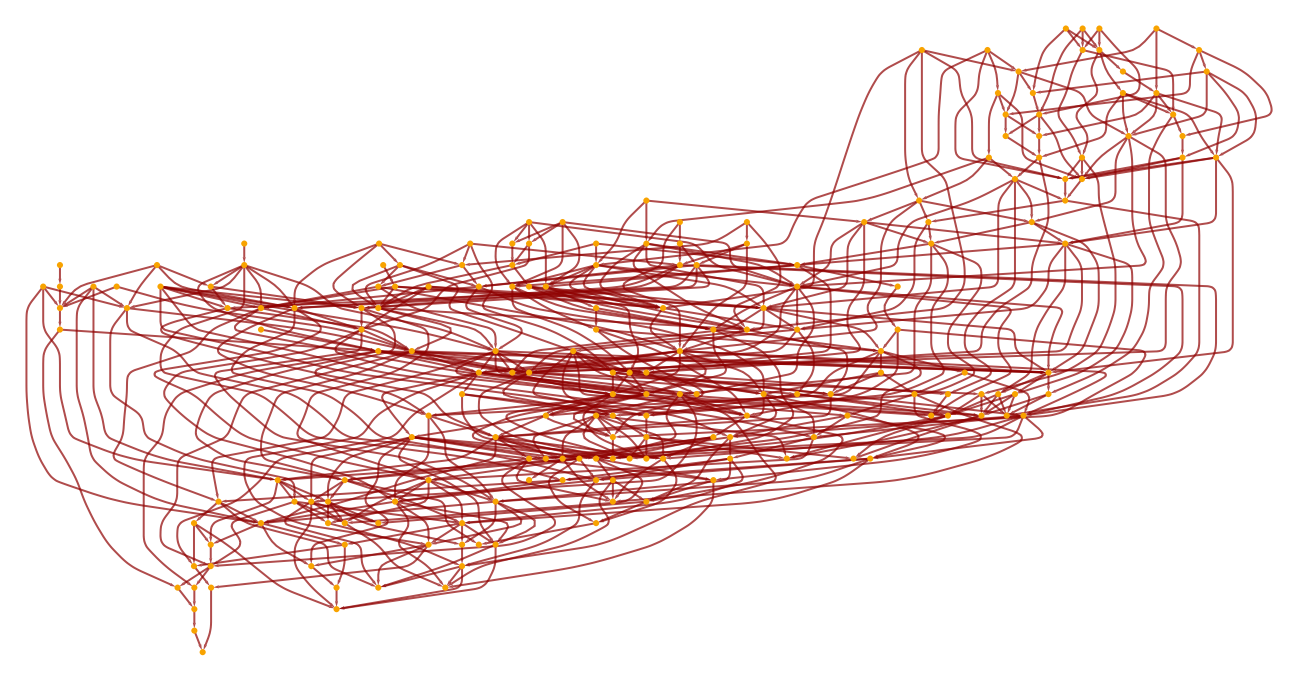A three-variable algebraic curvature function:

 In:=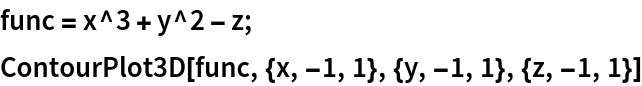Out=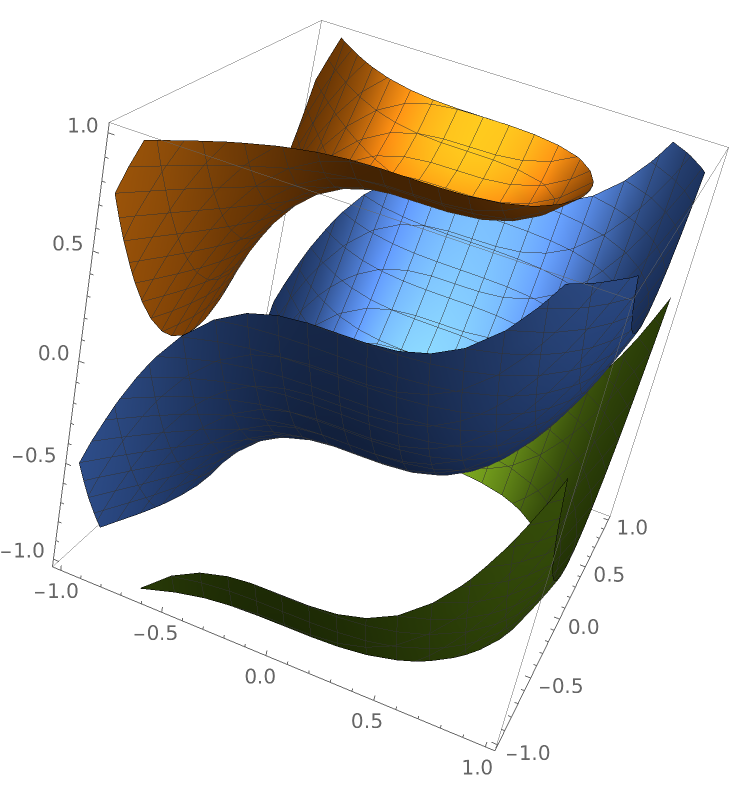Produce a random sprinkling of 200 points into a 2+1-dimensional spacetime with the specified curvature function:

 In:=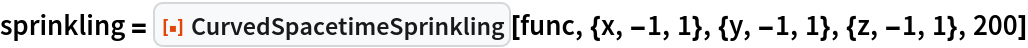Out=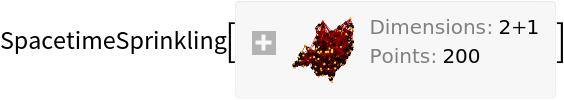Plot the positions of the sprinkled points only (without causal edges):

 In:=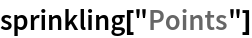Out=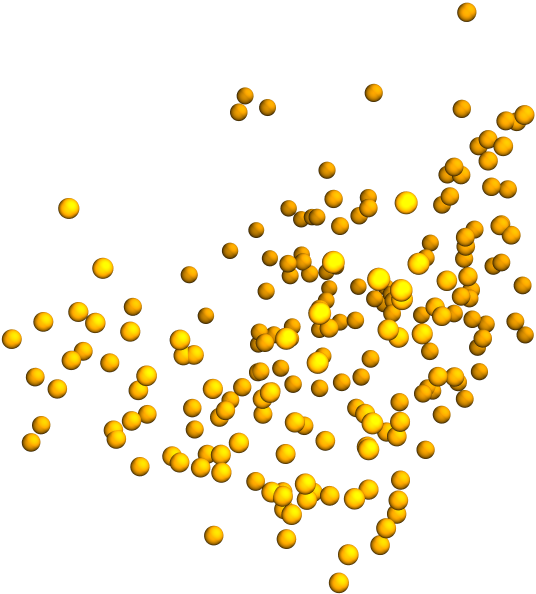Show the causal graph:

 In:=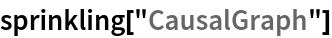Out=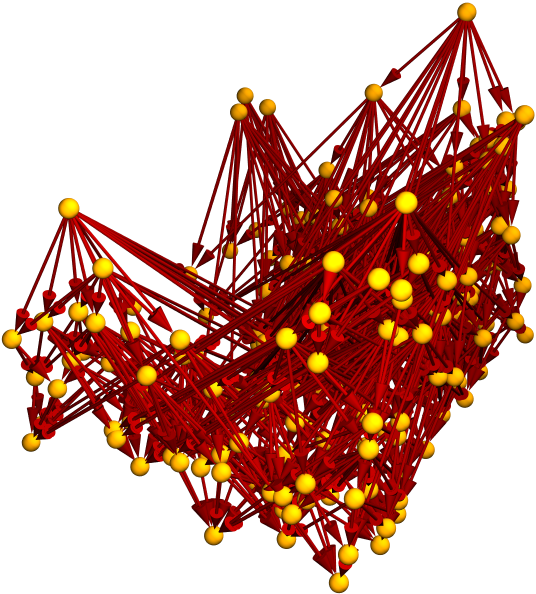### Scope (5)

Sprinklings can be produced in 0+1-dimensional spacetimes:

 In:=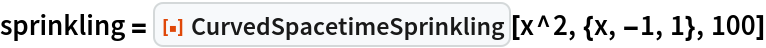Out=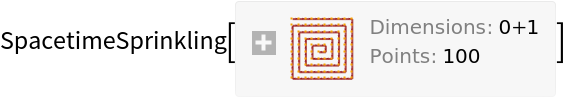Show the pure causal graph:

 In:=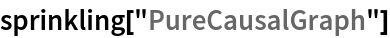Out=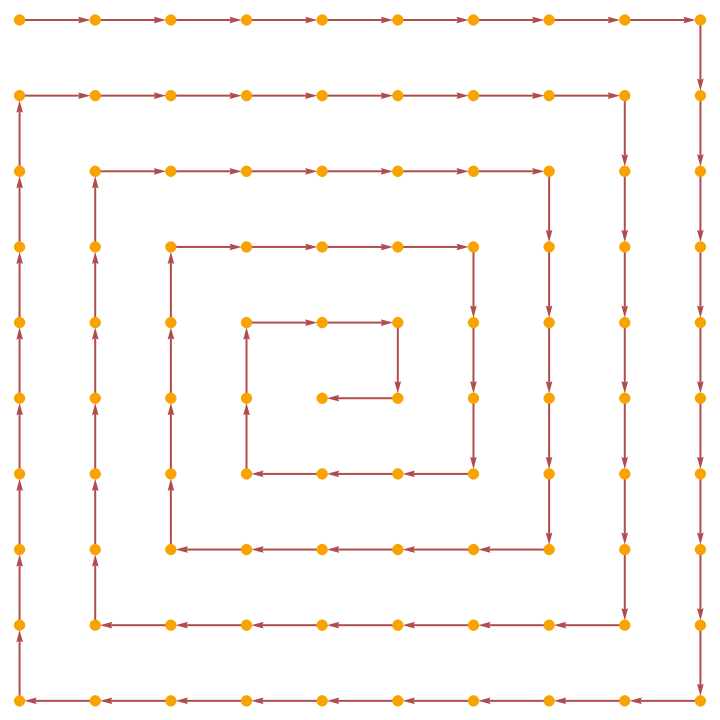A sprinkling in 1+1-dimensional spacetime:

 In:=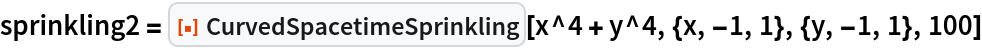Out=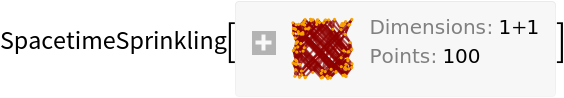Show the causal graph:

 In:=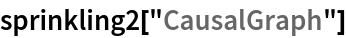Out=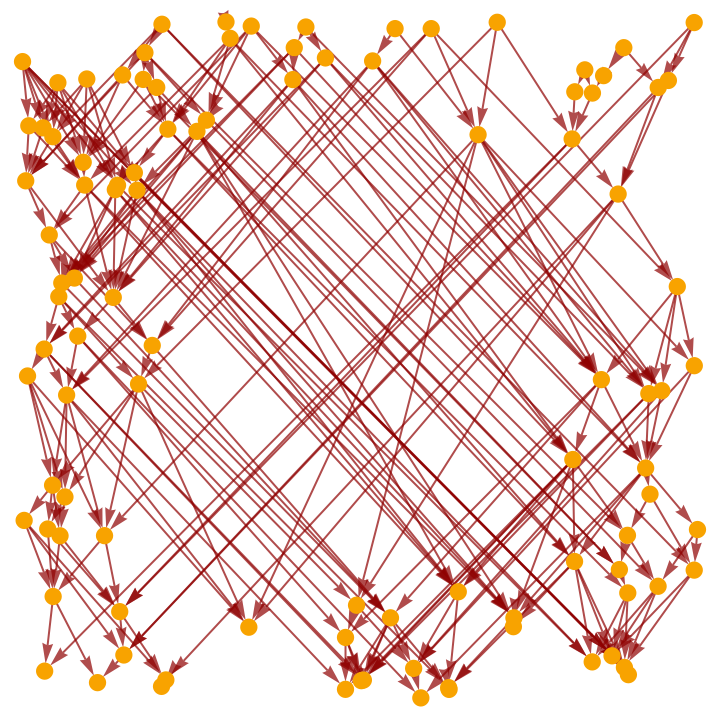A sprinkling in 2+1-dimensional spacetime:

 In:=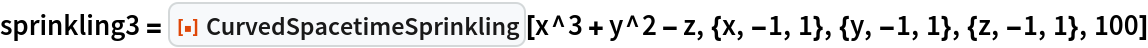Out=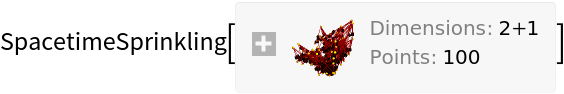Show the causal graph. Note that, unlike FlatSpacetimeSprinkling, CurvedSpacetimeSprinkling does not support higher-dimensional sprinklings:

 In:=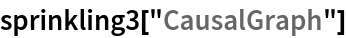Out=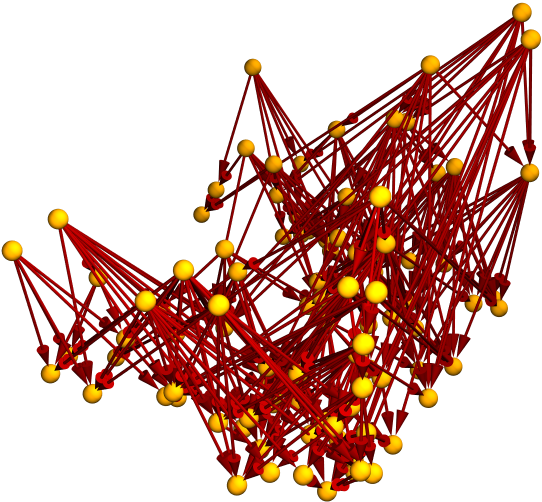Properties can be requested directly from CurvedSpacetimeSprinkling:

 In:=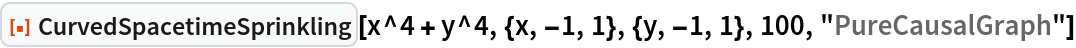Out=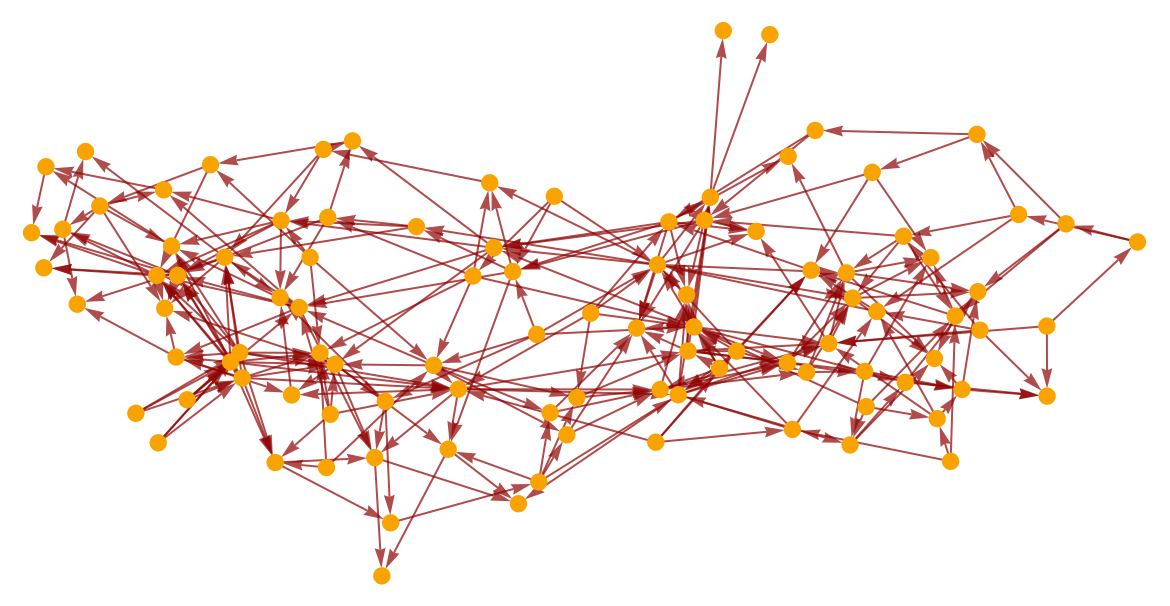Produce a random sprinkling of 100 points into a 1+1-dimensional spacetime with a complicated algebraic curvature function:

 In:=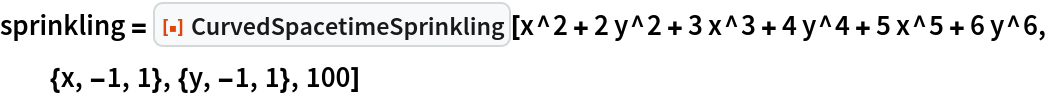Out=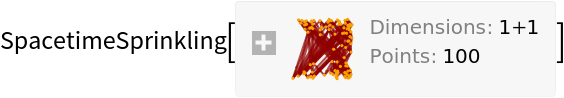Show the complete list of properties:

 In:=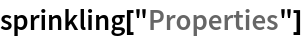Out=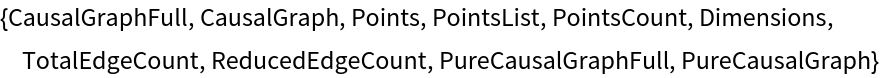Show the full causal graph:

 In:=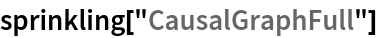Out=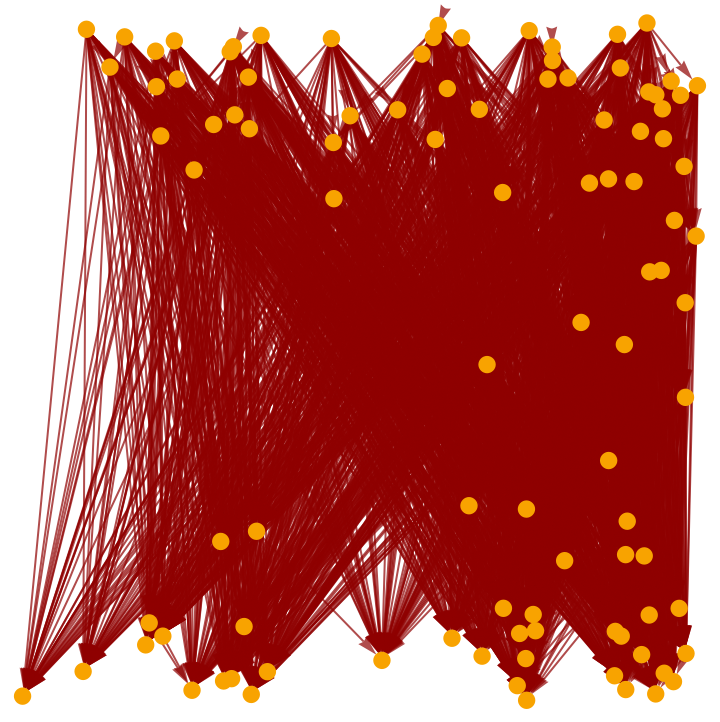Show the transitively reduced causal graph:

 In:=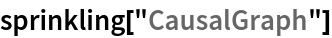Out=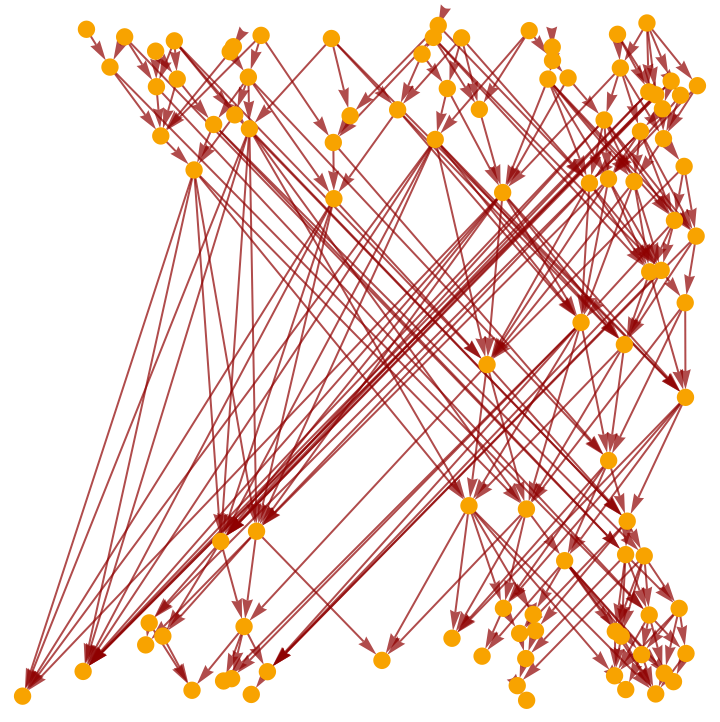Plot the positions of the sprinkled points only (without causal edges):

 In:=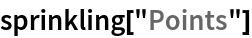Out=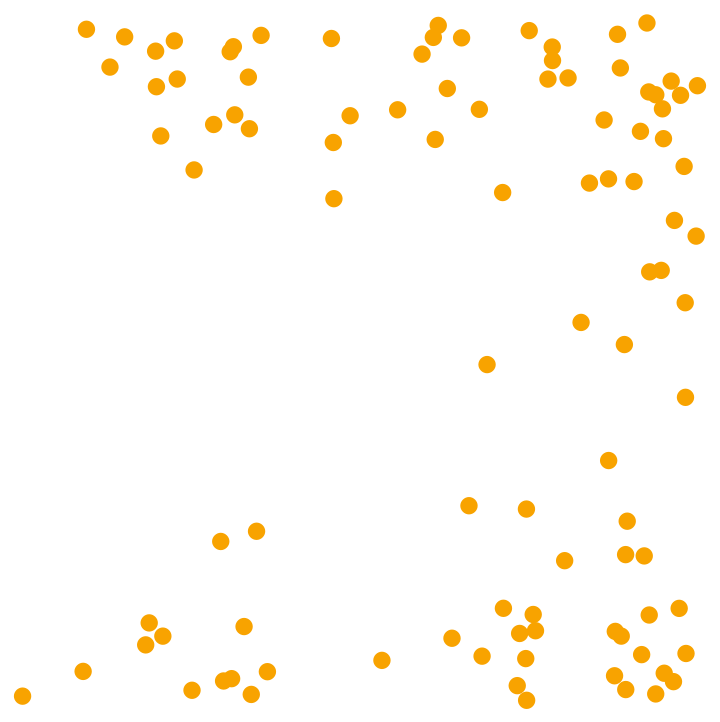Show the complete list of spacetime coordinates for the sprinkled points:

 In:=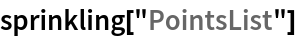Out=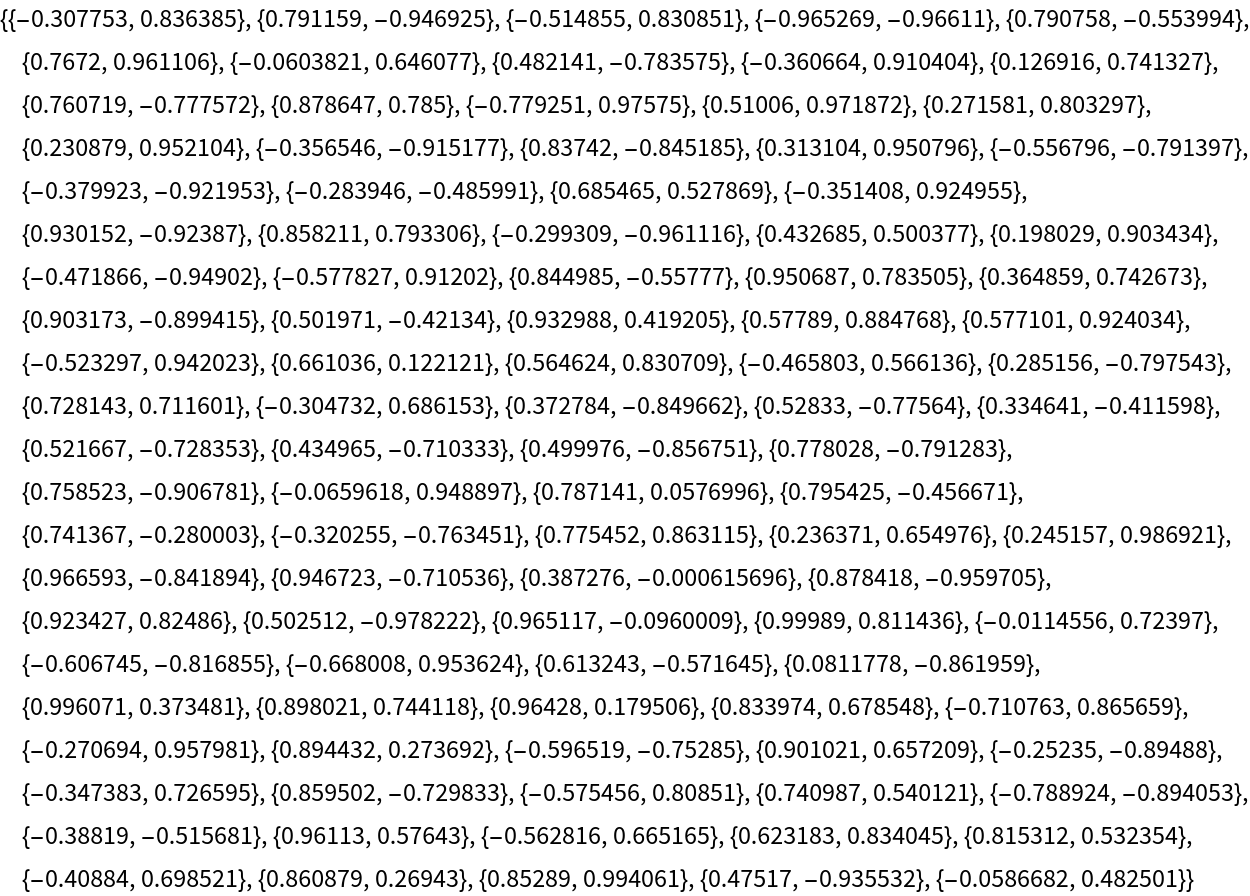Show the number of sprinkled points:

 In:=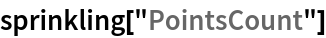Out=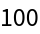Show the number of dimensions in the background spacetime:

 In:=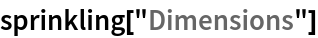Out=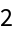Show the total number of causal edges:

 In:=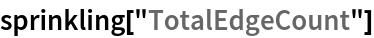Out=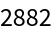Show the number of causal edges after transitive reduction:

 In:=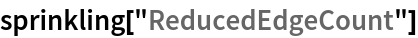Out=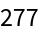Show the pure full causal graph (with vertex coordinate information removed):

 In:=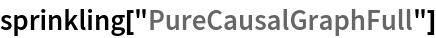Out=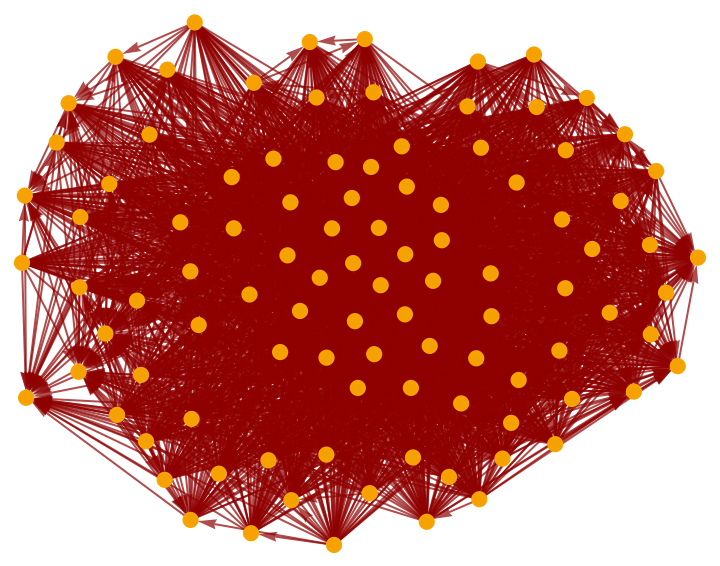Show the pure transitively reduced causal graph (with vertex coordinate information removed):

 In:=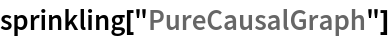Out=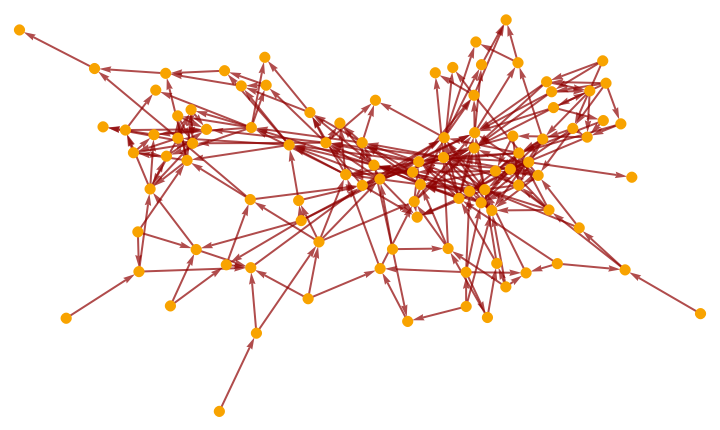Jonathan Gorard

## Version History

• 1.0.0 – 17 November 2020